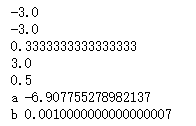﻿ Python幂运算与正负号 – 蒋智昊的博客# Python幂运算与正负号

```
#python 中  幂运算与正负号
#python 中  如果幂运算前有正负号，则 - + 的优先级会比**低。而当 - + 在后面时，优先级会比 ** 高。

import numpy as np

print(-9 ** 0.5)

print(-(9 ** 0.5))

print(9 ** -0.5) #变倒数

print(9 ** 0.5)

print(4 ** -0.5) #变倒数

print('a', np.log(0.001))
print('b', np.e ** -6.907755278982137)
```